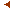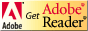this issueprevious article in this issue

 Document Details : Title: On the Nature of Discrete Space-Time Subtitle: Part 1: The Distance Formula, Relativistic Time Dilation and Length Contradiction in Discrete Space-Time Author(s): CROUSE, David , SKUFCA, Joseph Journal: Logique et Analyse Volume: 246    Date: 2019   Pages: 177-223 DOI: 10.2143/LEA.246.0.3286443 Abstract : In this work, the relativistic phenomena of Lorentz-Fitzgerald contraction and time dilation are derived using a modified distance formula that is appropriate for discrete space. This new distance formula is different than the Pythagorean theorem but converges to it for distances large relative to the Planck length. First, four candidate formulas developed by different people over the last 70 years are discussed. Three of the formulas are shown to be identical for conditions that best describe discrete space. It is shown that this new distance formula is valid for all size-scales, from the Planck length upwards, and solves two major problems historically associated with the discrete space-time (DST) model. One problem it solves is the widely believed anisotropic nature of most discrete space models. Just as commonly believed is the second problem: the incompatibility of DST's concept of an immutable atom of space and the length contraction of this atom required by special relativity. The new formula for the calculations of distance in DST solves this problem. It is shown that length contraction of the atom of space does not occur for any relative velocity of reference frames. It is also shown that time dilation of the atom of time does not occur. Also discussed is the possibility of any object being able to travel at the speed of light for specific temporal durations given by an equation derived in this work. Also discussed is a method to empirically verify the discreteness of space by studying any observed anomalies in the motion of astronomical bodies, such as differences in the bodies' inertial masses and gravitational masses. The importance of the new distance formula for causal set theory and other theories of quantum gravity is also discussed. download article3.228.11.9.